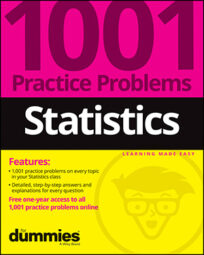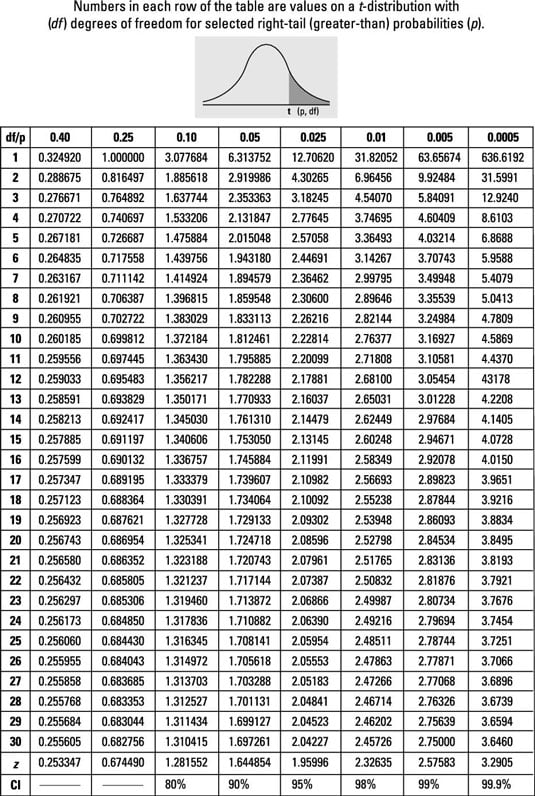##### Statistics: 1001 Practice Problems For Dummies (+ Free Online Practice)The t-table (for the t-distribution) is different from the z-table (for the z-distribution). Make sure you understand the values in the first and last rows. Finding probabilities for various t-distributions, using the t-table, is a valuable statistics skill.

## How to use the t-table to find right-tail probabilities and p-values for hypothesis tests involving t:

1. First, find the t-value for which you want the right-tail probability (call it t), and find the sample size (for example, n).
2. Next, find the row corresponding to the degrees of freedom (df) for your problem (for example, n – 1). Go across that row to find the two t-values between which your t falls. For example, if your t is 1.60 and your n is 7, you look in the row for df = 7 – 1 = 6. Across that row you find your t lies between t-values 1.44 and 1.94.
3. Then, go to the top of the columns containing the two t-values from Step 2. The right-tail (greater-than) probability for your t-value is somewhere between the two values at the top of these columns. For example, your t = 1.60 is between t-values 1.44 and 1.94 (df = 6); so the right tail probability for your t is between 0.10 (column heading for t = 1.44); and 0.05 (column heading for t = 1.94).

The row near the bottom with Z in the df column gives right-tail (greater-than) probabilities from the Z-distribution.

Use the t table to find t*-values (critical values) for a confidence interval involving t:

1. Determine the confidence level you need (as a percentage).
2. Determine the sample size (for example, n).
3. Look at the bottom row of the table where the percentages are shown. Find your % confidence level there.
4. Intersect this column with the row representing your degrees of freedom (df).
This is the t-value you need for your confidence interval. For example, a 95% confidence interval with df=6 has t*=2.45. (Find 95% on the last line and go up to row 6.)## Practice solving problems using the t-table sample questions below

1. For a study involving one population and a sample size of 18 (assuming you have a t-distribution), what row of the t-table will you use to find the right-tail (“greater than”) probability affiliated with the study results?

The study involving one population and a sample size of 18 has n – 1 = 18 – 1 = 17 degrees of freedom.

2. For a study involving a paired design with a total of 44 observations, with the results assuming a t-distribution, what row of the table will you use to find the probability affiliated with the study results?

A matched-pairs design with 44 total observations has 22 pairs. The degrees of freedom is one less than the number of pairs: n – 1 = 22 – 1 = 21.

3. A t-value of 2.35, from a t-distribution with 14 degrees of freedom, has an upper-tail (“greater than”) probability between which two values on the t-table?